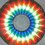# It's not a geometry problem this time ; - D

Let x be a real number such that $x^{ 2014} -x^{ 2004} and x^{ 2009 }-x^{ 2004}$ are both integers. Show that x is an integer.Note by Vishnu C
6 years, 2 months ago

This discussion board is a place to discuss our Daily Challenges and the math and science related to those challenges. Explanations are more than just a solution — they should explain the steps and thinking strategies that you used to obtain the solution. Comments should further the discussion of math and science.

When posting on Brilliant:

• Use the emojis to react to an explanation, whether you're congratulating a job well done , or just really confused .
• Ask specific questions about the challenge or the steps in somebody's explanation. Well-posed questions can add a lot to the discussion, but posting "I don't understand!" doesn't help anyone.
• Try to contribute something new to the discussion, whether it is an extension, generalization or other idea related to the challenge.

MarkdownAppears as
*italics* or _italics_ italics
**bold** or __bold__ bold
- bulleted- list
• bulleted
• list
1. numbered2. list
1. numbered
2. list
Note: you must add a full line of space before and after lists for them to show up correctly
paragraph 1paragraph 2

paragraph 1

paragraph 2

[example link](https://brilliant.org)example link
> This is a quote
This is a quote
    # I indented these lines
# 4 spaces, and now they show
# up as a code block.

print "hello world"
# I indented these lines
# 4 spaces, and now they show
# up as a code block.

print "hello world"
MathAppears as
Remember to wrap math in $$ ... $$ or $ ... $ to ensure proper formatting.
2 \times 3 $2 \times 3$
2^{34} $2^{34}$
a_{i-1} $a_{i-1}$
\frac{2}{3} $\frac{2}{3}$
\sqrt{2} $\sqrt{2}$
\sum_{i=1}^3 $\sum_{i=1}^3$
\sin \theta $\sin \theta$
\boxed{123} $\boxed{123}$

Sort by:

Fixing the solution of Visnhu C.

Subtract the second from the first and you'll get that $x^5(x^{2009}-x^{2004})$ is an integer

We see that $x^5$ is a rational number. That implies $x^5 - 1$ is also a rational number.

Now, $x^{2009} - x^{2004} = x^{2004}(x^5 - 1) = (x^5)^{400}*x^4*(x^5 - 1)$ implies that $x^4$ is also a rational number. Therefore $\dfrac{x^5}{x^4} = x$ is also a rational number.

Now assume $x = \frac{p}{q}$ where $p,q$ are co-prime integers. Then $x^{2009} - x^{2004} = x^{2004}(x^5 - 1) = \frac{p^{2004}}{q^{2004}}(\frac{p^5}{q^5} - 1)$

$= \frac{p^{2004}}{q^{2004}}( \frac{p^5 - q^5}{q^5}) = \frac{p^{2004}(p^5 - q^5)}{q^{2014}}$

Now, we see that $p^5 - q^5 \equiv p^5 \not \equiv 0 \pmod{q}$. So $q$ does not divide either $p^{2004}$ or $(p^5 - q^5)$,. Therefore the rational number is in its lowest terms and its denominator is $q^{2014}$. But since $x^{2009} - x^{2004}$ is an integer, $q^{2014} = 1 \implies q = 1$. Therefore $x$ is an integer.

Also, vishnu c , is this a question from this year's paper? If so, could you post all the questions here?

- 6 years, 2 months ago

Once you know that x is rational, you can just use the rational root theorem.

- 6 years, 2 months ago

Oh. I was unaware of that form of the rational root theorem. That makes my solution smaller. Thanks.

and TBH, my solution was small on paper. Writing down the justifications and calculations made it seem much bigger than it should have been.

- 6 years, 2 months ago

Thanks man! I'm sorry for the mistake! Learnt my lesson.

- 6 years, 2 months ago

Oh, I also shared a set with CMI's questions form last year's paper. This one is also from last year's paper. I'm gonna be writing this year's paper on Monday! Wish me luck! I hope I don't make this kind of mistakes in the hall.

- 6 years, 2 months ago

The rational root theorem states that when you have a polynomial with nonzero leading coefficient and constant term, like $x^5-1$ then every rational x that is a root of this polynomial, expressed as p/q, where p and q are coprime integers satisfies these two conditions:

• p divides the constant term and,
• q divides the leading coefficient.

In our case, we have the equation $x^{2014}-x^{2004}=k, \quad \forall \quad k\in Z.$ So, we get that q divides 1 and p divides any integer you throw at it. So, we get that x is an integer.

- 6 years, 2 months ago

Again, you have to be careful: We don't know at this point that $x^5-1$ is an integer.

- 6 years, 2 months ago

It's the middle of the night here. I can't see clearly. Good night!

- 6 years, 2 months ago

Ok good night... to be continued. You want to apply the rational root theorem to one of the original equations, for example, $x^{2014}-x^{2004}-k=0$, since we are told that $k$ is an integer.

- 6 years, 2 months ago